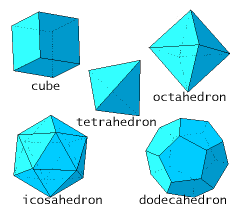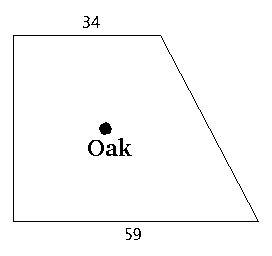Logic puzzles, riddles, math puzzles and brainteasers - pzzls.com

Vandaag is het 1 October 2022

Sort - Least popuplar on top
Page: << 6 7 8 9 10 >>

# Number magic - math puzzle

Difficulty:Rating: 2.8/5.0

If you multiply me by 2, subtract 1, and read the reverse the result you'll find me. Which numbers can I be?

# Platonic solids - math puzzle

Difficulty:Rating: 2.8/5.0

 Show that there exist only five platonic solids (that are objects which are build out of the one sort of regular polygons, like the tetrahedron and the cube). Hint: use that for each solid in three dimensions it holds that the number of vertices + the number of faces is equal to the number of edges + 2.# The walled garden - math puzzle

Difficulty:Rating: 2.8/5.0

 A garden is surrounded by four walls, which form a rectangular trapezoid. The parallel walls have lengths of 34 and 59. An old oak grows in the middle of the garden. The owner of the garden has figured out that the distance from the four walls to the oak are exactly the same. What is the area of the garden?